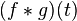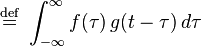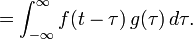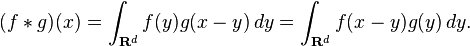# A C Program to Find Convolution of Two Signals

The convolution of ƒ and g is written ƒg, using an asterisk or star. It is defined as the integral of the product of the two functions after one is reversed and shifted. As such, it is a particular kind of integral transform:(commutativity)

While the symbol t is used above, it need not represent the time domain. But in that context, the convolution formula can be described as a weighted average of the function ƒ(τ) at the moment t where the weighting is given by g(−τ) simply shifted by amount t. As t changes, the weighting function emphasizes different parts of the input function.

More generally, if f and g are complex-valued functions on Rd, then their convolution may be defined as the integral:In Digital Signal Processing where convolution is done between two discrete signals the procedure followed is :There are different methods used in finding convolution (refer Signals And Systems by  Openheim for more details on each method). Here i will demonstrate a C program that uses the matrix method to find convolution.
The CODE
/* Vineeth Kartha
Program To Find Convolution
Released Under GPL */
#include<stdio.h>
int main()
{
printf(“\n\tThis program finds the convolution of a signal and its Impulse response\n”);
printf(“—————————————————————————\n\n”);
int i,j,k,x,h,y,a,n,m;
printf(“enter the number of elements in x[n]:\t”);
scanf(“%d”,&n);
printf(“enter the elements of x[n]:\n”);
for(i=0;i<n;i++)
{
scanf(“%d”,&x[i]);
}
printf(“enter the number of elements in h[n]:\t”);
scanf(“%d”,&m);
printf(“enter the elements of h[n]:\n”);
for(i=0;i<m;i++)
{
scanf(“%d”,&h[i]);
}
for(k=0;k<m;k++)
{
for(i=0;i<n;i++)
{
a[k][i]=h[k]*x[i];
}
}
for(k=0;k<(n+m-1);k++)
{
y[k]=0;
for(i=0;i<m;i++)
{
for(j=0;j<n;j++)
{
if((i+j)==k)
{
y[k]+=a[i][j];
}
}
}
}
printf(“\n\tx[n]={“);
for(i=0;i<n;i++)
{
printf(“%d “,x[i]);
}
printf(“}\n\th[n]={“);
for(i=0;i<m;i++)
{
printf(“%d “,h[i]);
}printf(“}\n\n\n\ty[n]=x[n]*h[n]\n——————————————\n\n\ty[n]={“);for(i=0;i<(n+m-1);i++)
{
printf(“%d “,y[i]);
}
printf(“}\n\n”);
return 0;
}

## 8 thoughts on “A C Program to Find Convolution of Two Signals”

1.онлайн казино

A person necessarily lend a hand to make seriously articles
I might state. That is the very first time I frequented your
web page and thus far? I surprised with the analysis you made to
make this actual submit amazing. Wonderful job!

2. You really make it seem so easy with your presentation but I find this matter to be really something that I think I would never understand.
It seems too complicated and very broad for me. I’m
looking forward for your next post, I will try to get the
hang of it!

3.Chemist Direct

After looking ovedr a number of the articles
blog. I book-marked it tto my bookmark website list and will be checking back soon. Please visit mmy
web site too and tepl mme how you feel.

4.nisim

Hi there too all, how is the whole thing, I think every oone is getting more from this
site, and your views aree good in favor oof new viewers.

5.Wilbur

I do accept as true with all the ideas you have introduced to your post.
They’re vedy convincing and will certainly work.
Nonetheless, the posts are very short for beginners.

May jut you please lengthen them a bit frrom subsequent
time? Thank you for the post.

6.raquela

Why this code didn’t work? I have a lot of mistakes during compilations

1.vineethkartha

1.raquela